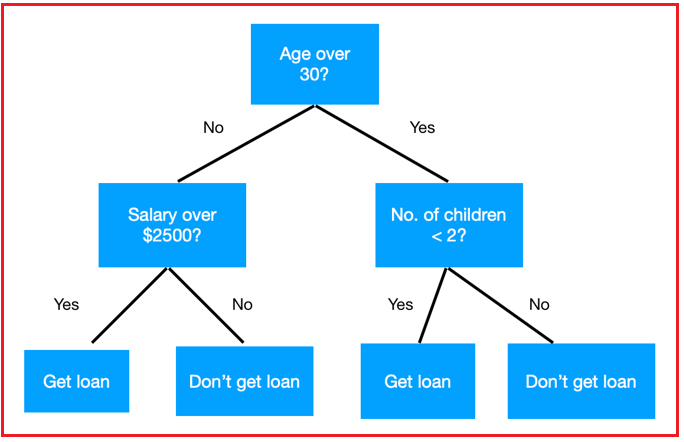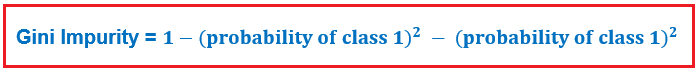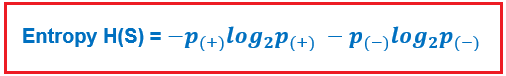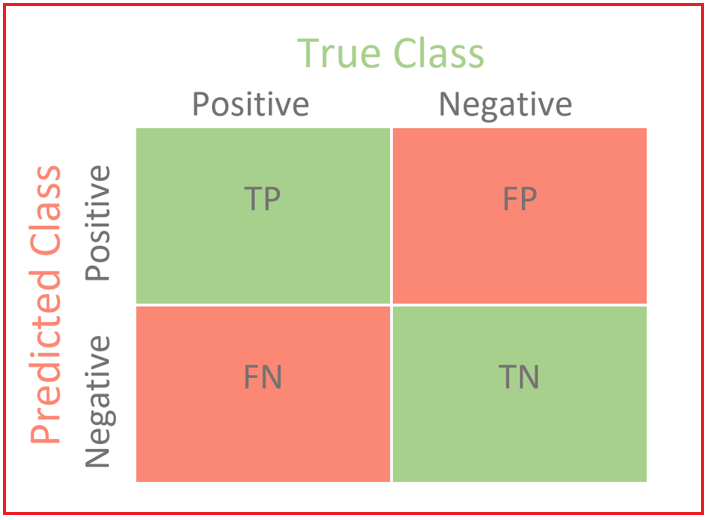# Decision Tree in Machine Learning

## Decision Tree in Machine Learning

In this article, I am going to discuss the Decision Tree in Machine Learning with Examples. Please read our previous article where we discussed Classification and its Use Cases in Machine Learning with Examples.

##### What is a Decision Tree in Machine Learning?

A Decision Tree is a basic diagram for categorizing examples. It’s supervised machine learning in which data is constantly separated according to a parameter. The following are the components of a decision tree:

1. Nodes: Check the value of a certain attribute.
2. Edges/Branches: Connect to the next node or leaf based on the results of a test.
3. Leaf Nodes: Terminal nodes that forecast the outcome are known as leaf nodes (represent class labels or class distribution).Consider the example above to grasp the notion of a Decision Tree. Let’s imagine you want to know whether a person will get a loan based on the salary, number of children, and other factors. ‘What is his age?’, ‘What’s his salary, and ‘How many children does a person have?’ are examples of decision nodes. The outcomes – ‘Get Loan’, and ‘Doesn’t Get Loan’ are represented by leaf nodes. There are two types of decision trees –

1. Regression Tree
2. Classification Tree
##### Decision Tree Classifier in Machine Learning

In cases where the outcome type of a decision tree is categorical or discrete, we call it a Decision Tree Classifier. Example – predicting whether a person will get a loan or not.

A procedure called binary recursive partitioning is used to create such a tree. This is an iterative method that involves separating the data into partitions and then further splitting it up on each branch.

This method is also known as divide and conquer because it divides the data into subsets, which are then divided again into even smaller subsets, and so on until the algorithm concludes that the data inside the subgroups is sufficiently homogeneous, or another stopping requirement is reached. The Divide-and-Conquer Algorithm is a basic divide-and-conquer strategy.

1. Choose a root node test. Create a branch for each potential test result.
2. Subdivide instances into groups. Each branch extending from the node has its own.
3. Recursively repeat for each branch, only using instances that reach the branch.
4. If all of a branch’s instances share the same class, the recursion should be stopped.
##### How to create a Decision Tree in Machine Learning?

A decision tree can be built using a variety of algorithms. These algorithms are based on the type of dataset and the type of impurity measure used. Let’s have a look at these algorithms –

##### CART (Classification and Regression Trees)

CART is a deep learning technique that generates binary Classification or Regression Trees depending on whether the dependent (or target) variable is categorical or numeric. It works with raw data (no preprocessing required) and can employ the same variables multiple times in the same DT, potentially revealing intricate interdependencies across groups of variables.

We need a way to quantify and compare impurity to determine which separation is optimal. The Gini impurity score is the metric used in the CART method to measure impurity. Gini impurity is simple to calculate. The final split should be the one with the least Gini impurity. Let’s have a look at the mathematical formula of Gini Impurity –So, the following are the steps to create a decision tree –

1. Calculate the Gini scores for impurities for each possible split using different features.
2. If the node has the lowest score, then it becomes a leaf node.
3. If data separation improves performance, choose the separation with the lowest impurity value.
##### ID3-Iterative Dichotomiser 3

Except for the method for detecting purity/impurity, the process of generating a decision tree using the ID3 algorithm is nearly identical to that of using the CART algorithm.

Entropy is the statistic used in the ID3 algorithm to determine purity. Entropy is a measure of a class’s uncertainty in a subset of examples. Assume that the item belongs to the S subset, which has two classes: positive and negative. The number of bits required to determine whether x is positive or negative is known as entropy.Where,

= % of positive examples in subset S

= % of negative examples in subset S

The entropy value is always between 0 and 1. So, if a subset generated after an attribute separation is pure, we’ll need zero bits to determine if it’s positive or negative.

##### Confusion Matrix in Machine Learning

The Confusion Matrix is one of the most natural and simple (unless you’re not confused) measures for determining the model’s correctness and accuracy. It’s used to solve classification problems where the output can be divided into two or more classes.

Let’s pretend we’re working on a classification problem that requires us to predict whether or not someone has cancer. Let’s give our target variable a label:

1: When a person is diagnosed with cancer.

0: When someone does not have cancer.

The confusion matrix is a two-dimensional table with sets of “classes” in both dimensions (“Actual” and “Predicted”). Columns represent actual classifications, whereas rows represent predicted classifications.Although the Confusion Matrix and the values within it are not a performance measure in and of itself, they are the foundation for practically all performance measurements. Now, let’s understand the terms related to the confusion matrix –

• True Positives: True positives occur when the data point’s actual class is 1 (True) and the projected class is also 1. (True). Example – True positive describes the situation in which a person has cancer(1) and the model classifies his case as cancer(1).
• True Negatives: True negatives occur when the data point’s real class is 0 (False) and the anticipated value is also 0. (False). Example – True Negatives refers to the situation in which a person does not have cancer yet the model classifies his condition as such.
• False Positives: False positives occur when the data point’s real class is 0 (False) but the projected class is 1. (True). Example – False Positives are when a person does not have cancer but the model classifies his case as cancer.
• False Negatives: False negatives occur when the data point’s real class is 1 (True) but the anticipated value is 0. (False). Example – False Negatives are when a person has cancer and the model classifies his condition as non-cancer.

The ideal case is for the model to produce zero false positives and zero false negatives. However, this is not the case in real life, as no model can ever be completely correct.

##### What should be minimized when?

We know that every model we employ to forecast the true class of the target variable will have some error. False Positives and False Negatives will happen as a result of this (i.e Model classifying things incorrectly as compared to the actual class).

There is no hard and fast rule that states what should be minimized in every circumstance. It is entirely dependent on the business requirements and the context of the problem to be solved. As a result, we may desire to reduce the number of False Positives or False Negatives.

##### Minimizing False Negatives –

In our cancer detection problem, let’s presume that only 5 people out of 100 have cancer. We want to correctly categorize all malignant patients in this situation because even a very BAD model (predicting everyone as nON-Cancerous) will give us a 95 percent accuracy (will come to what accuracy is).

However, in attempting to catch all cancer cases, we may wind up classifying someone who is not genuinely suffering from cancer as cancerous. This may be acceptable because it is less risky than failing to identify/capture a cancerous patient, as we will be sending the cancer cases for additional inspection and reports anyhow. However, missing a cancer patient would be a tremendous mistake because they would not be examined further.

##### Minimizing False Positives –

Let’s look at a second scenario where the model identifies whether an email is a spam or not to better understand False Positives. Assume you’re expecting a crucial email, such as a response from a recruiter or an acceptance letter from a university.

Let’s say the Model classifies that crucial email you’ve been waiting for as spam (case of False positive). In this scenario, this is worse than labeling a spam email as essential or not important because we can still manually remove it and it’s not a big deal if it happens once in a while. As a result, when it comes to spam email classification, reducing false positives is more important than reducing false negatives.

In the next article, I am going to discuss Model Evaluation for Classification in Machine Learning with Examples. Here, in this article, I try to explain the Decision Tree in Machine Learning with Examples. I hope you enjoy this Decision Tree in Machine Learning with Examples article.